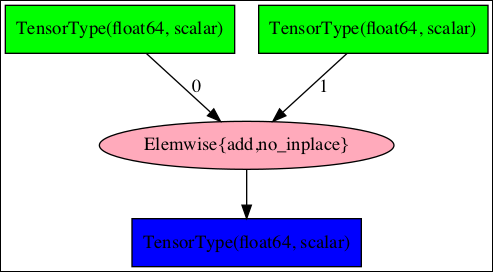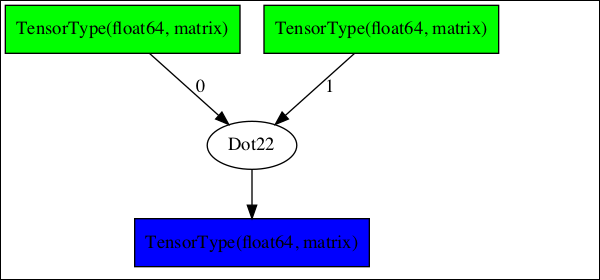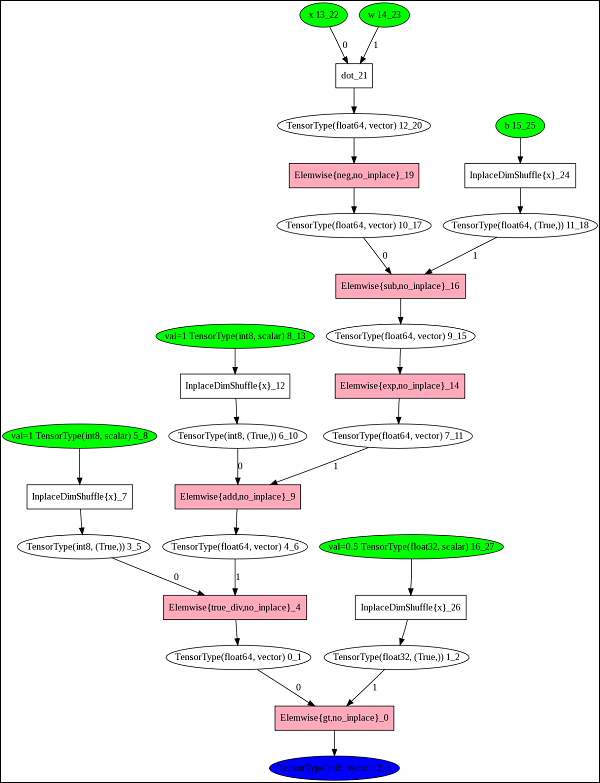# Theano - Computational Graph

From the above two examples, you may have noticed that in Theano we create an expression which is eventually evaluated using the Theano function. Theano uses advanced optimization techniques to optimize the execution of an expression. To visualize the computation graph, Theano provides a printing package in its library.

## Symbolic Graph for Scalar Addition

To see the computation graph for our scalar addition program, use the printing library as follows −

theano.printing.pydotprint(f, outfile="scalar_addition.png", var_with_name_simple=True)


When you execute this statement, a file called scalar_addition.png will be created on your machine. The saved computation graph is displayed here for your quick reference −The complete program listing to generate the above image is given below −

from theano import *
a = tensor.dscalar()
b = tensor.dscalar()
c = a + b
f = theano.function([a,b], c)


## Symbolic Graph for Matrix Multiplier

Now, try creating the computation graph for our matrix multiplier. The complete listing for generating this graph is given below −

from theano import *
a = tensor.dmatrix()
b = tensor.dmatrix()
c = tensor.dot(a,b)
f = theano.function([a,b], c)
theano.printing.pydotprint(f, outfile="matrix_dot_product.png", var_with_name_simple=True)


The generated graph is shown here −## Complex Graphs

In larger expressions, the computational graphs could be very complex. One such graph taken from Theano documentation is shown here −To understand the working of Theano, it is important to first know the significance of these computational graphs. With this understanding, we shall know the importance of Theano.

## Why Theano?

By looking at the complexity of the computational graphs, you will now be able to understand the purpose behind developing Theano. A typical compiler would provide local optimizations in the program as it never looks at the entire computation as a single unit.

Theano implements very advanced optimization techniques to optimize the full computational graph. It combines the aspects of Algebra with aspects of an optimizing compiler. A part of the graph may be compiled into C-language code. For repeated calculations, the evaluation speed is critical and Theano meets this purpose by generating a very efficient code.